# Venn Diagrams on R Studio

### First step: Install & load “VennDiagram” package.

``````# install.packages('VennDiagram')
library(VennDiagram)
``````

Add filepath if “catdoge.csv” is not in working-directory.

``````d <- read.csv("catdoge.csv")
``````

#### Creating subsets

We'll need these totals to plug into the venn diagram function

``````## areas of each animal
nrow(subset(d, Dog == 1))
``````
``````##  22
``````
``````nrow(subset(d, Cat == 1))
``````
``````##  20
``````
``````nrow(subset(d, Snake == 1))
``````
``````##  6
``````
``````nrow(subset(d, Lizard == 1))
``````
``````##  13
``````
``````
## areas of 2-group overlap

## dogs and cats
nrow(subset(d, Dog == 1 & Cat == 1))
``````
``````##  11
``````
``````
## dogs and lizards
nrow(subset(d, Dog == 1 & Lizard == 1))
``````
``````##  5
``````
``````
## cats and lizards
nrow(subset(d, Cat == 1 & Lizard == 1))
``````
``````##  4
``````
``````
## lizards and snakes
nrow(subset(d, Lizard == 1 & Snake == 1))
``````
``````##  2
``````
``````
## 3 group overlap: dogs, cats and lizards
nrow(subset(d, Dog == 1 & Cat == 1 & Lizard == 1))
``````
``````##  1
``````

### Creating a Venn Diagram with a single circle

The basics

``````draw.single.venn(area = 22, category = "Dog People")
``````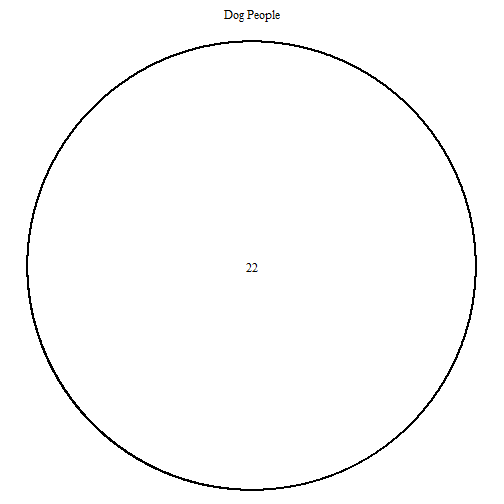``````## (polygon[GRID.polygon.4393], polygon[GRID.polygon.4394], text[GRID.text.4395], text[GRID.text.4396])
``````

Adding colour & removing the outline

``````## lty - outline of cirlces

## fill - colour

## alpha - colour transparency

grid.newpage()
draw.single.venn(22, category = "Dog People", lty = "blank", fill = "cornflower blue",
alpha = 0.5)
``````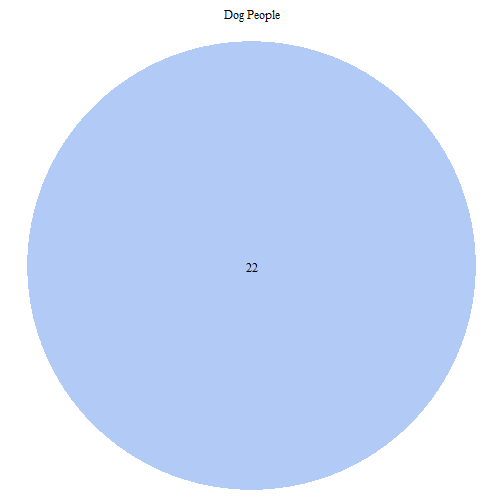``````## (polygon[GRID.polygon.4397], polygon[GRID.polygon.4398], text[GRID.text.4399], text[GRID.text.4400])
``````

### Creating a Venn Diagram with two circles

The basics. Note that circles are automatically scaled.

``````grid.newpage()
draw.pairwise.venn(area1 = 22, area2 = 20, cross.area = 11, category = c("Dog People",
"Cat People"))
``````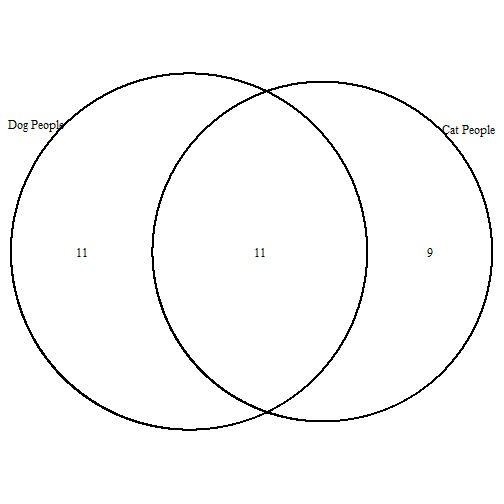``````## (polygon[GRID.polygon.4401], polygon[GRID.polygon.4402], polygon[GRID.polygon.4403], polygon[GRID.polygon.4404], text[GRID.text.4405], text[GRID.text.4406], text[GRID.text.4407], text[GRID.text.4408], text[GRID.text.4409])
``````

``````## cat.pos - position of category titles, represented by degree from the
## middle of the circle

## cat.dist - distance of the category titles from the edge of the circle

grid.newpage()
draw.pairwise.venn(22, 20, 11, category = c("Dog People", "Cat People"), lty = rep("blank",
2), fill = c("light blue", "pink"), alpha = rep(0.5, 2), cat.pos = c(0,
0), cat.dist = rep(0.025, 2))
``````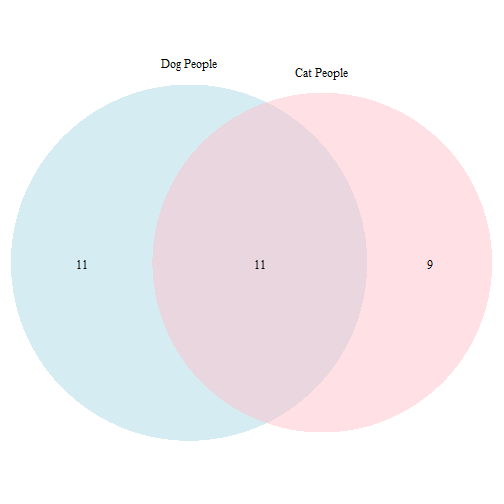``````## (polygon[GRID.polygon.4410], polygon[GRID.polygon.4411], polygon[GRID.polygon.4412], polygon[GRID.polygon.4413], text[GRID.text.4414], text[GRID.text.4415], text[GRID.text.4416], text[GRID.text.4417], text[GRID.text.4418])
``````

How to remove scaling

``````## scaled - TRUE for scaled or FALSE for unscaled cirlces

grid.newpage()
draw.pairwise.venn(22, 20, 11, category = c("Dog People", "Cat People"), lty = rep("blank",
2), fill = c("light blue", "pink"), alpha = rep(0.5, 2), cat.pos = c(0,
0), cat.dist = rep(0.025, 2), scaled = FALSE)
``````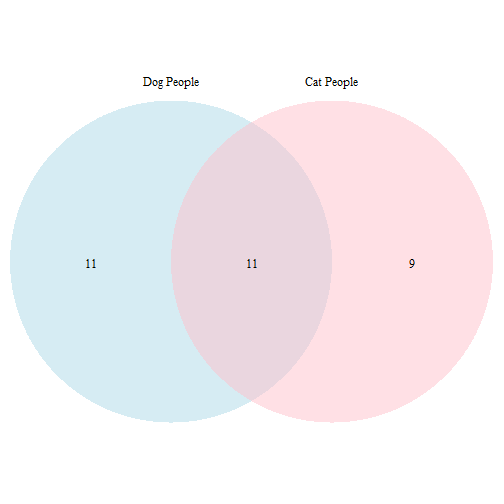``````## (polygon[GRID.polygon.4419], polygon[GRID.polygon.4420], polygon[GRID.polygon.4421], polygon[GRID.polygon.4422], text[GRID.text.4423], text[GRID.text.4424], text[GRID.text.4425], text[GRID.text.4426], text[GRID.text.4427])
``````

How to make two non-overlapping circles

``````## euler.d - TRUE for movable circles; FALSE for unmovable circles. Must be
## TRUE to have space between non-overlapping circles.

## sep.dist - distance between circles

## rotation.degree - degrees the diagram is rotated

grid.newpage()
draw.pairwise.venn(area1 = 22, area2 = 6, cross.area = 0, category = c("Dog People",
"Snake People"), lty = rep("blank", 2), fill = c("light blue", "green"),
alpha = rep(0.5, 2), cat.pos = c(0, 180), euler.d = TRUE, sep.dist = 0.03,
rotation.degree = 45)
``````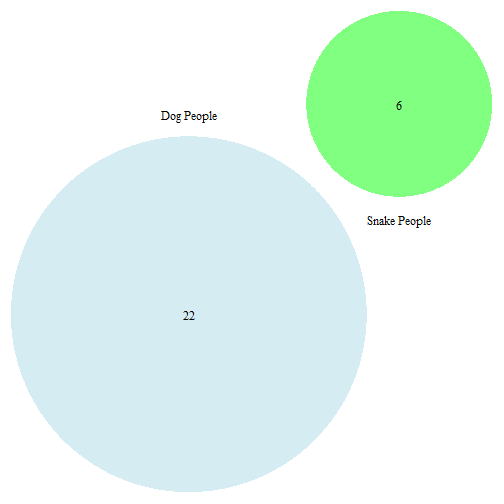``````## (polygon[GRID.polygon.4428], polygon[GRID.polygon.4429], polygon[GRID.polygon.4430], polygon[GRID.polygon.4431], text[GRID.text.4432], text[GRID.text.4433], text[GRID.text.4434], text[GRID.text.4435])
``````

### Creating a Venn Diagram with three circles

``````grid.newpage()
draw.triple.venn(area1 = 22, area2 = 20, area3 = 13, n12 = 11, n23 = 4, n13 = 5,
n123 = 1, category = c("Dog People", "Cat People", "Lizard People"), lty = "blank",
fill = c("skyblue", "pink1", "mediumorchid"))
``````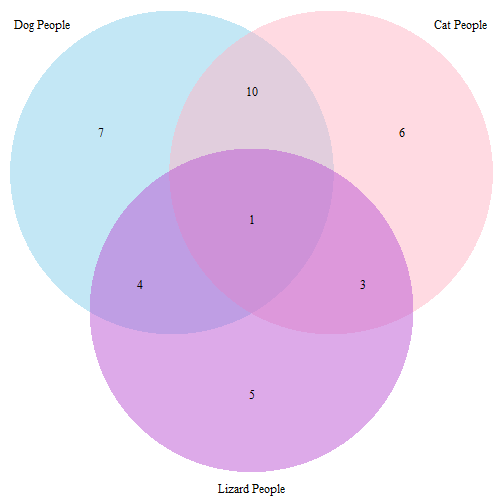``````## (polygon[GRID.polygon.4436], polygon[GRID.polygon.4437], polygon[GRID.polygon.4438], polygon[GRID.polygon.4439], polygon[GRID.polygon.4440], polygon[GRID.polygon.4441], text[GRID.text.4442], text[GRID.text.4443], text[GRID.text.4444], text[GRID.text.4445], text[GRID.text.4446], text[GRID.text.4447], text[GRID.text.4448], text[GRID.text.4449], text[GRID.text.4450], text[GRID.text.4451])
``````

### Using the subset function to pull values for the Venn Diagram

``````grid.newpage()
draw.triple.venn(area1 = nrow(subset(d, Dog == 1)), area2 = nrow(subset(d, Cat ==
1)), area3 = nrow(subset(d, Lizard == 1)), n12 = nrow(subset(d, Dog == 1 &
Cat == 1)), n23 = nrow(subset(d, Cat == 1 & Lizard == 1)), n13 = nrow(subset(d,
Dog == 1 & Lizard == 1)), n123 = nrow(subset(d, Dog == 1 & Cat == 1 & Lizard ==
1)), category = c("Dog People", "Cat People", "Lizard People"), lty = "blank",
fill = c("skyblue", "pink1", "mediumorchid"))
````````````## (polygon[GRID.polygon.4452], polygon[GRID.polygon.4453], polygon[GRID.polygon.4454], polygon[GRID.polygon.4455], polygon[GRID.polygon.4456], polygon[GRID.polygon.4457], text[GRID.text.4458], text[GRID.text.4459], text[GRID.text.4460], text[GRID.text.4461], text[GRID.text.4462], text[GRID.text.4463], text[GRID.text.4464], text[GRID.text.4465], text[GRID.text.4466], text[GRID.text.4467])
``````

## More stuff we can do with R programming and another package:

### Functions

Let's speed up the nrow(subset(…)) process for the area counts.
This “likes” function finds the total area for a circle or overlap subset, how many people like those animals. It takes the first letter(s) of the animal(s) in lower case, e.g. c(“d”, “c”)

``````likes <- function(animals) {
ppl <- d
names(ppl) <- c("p", "d", "c", "s", "l")
for (i in 1:length(animals)) {
ppl <- subset(ppl, ppl[animals[i]] == T)
}
nrow(ppl)
}

# How many people like dogs?
likes("d")
``````
``````##  22
``````
``````
# How many people in the dog-cat or cat-dog overlap?
likes(c("d", "c"))
``````
``````##  11
``````
``````likes(c("c", "d"))
``````
``````##  11
``````

Let's make one function that can call any of the venn diagram functions (pairwise, triple, etc).
This plotAnimals function can take up to 4 animals as c(“d”, “c”, “s”, “l”), and it will call the right venn diagram function and fill in the areas. It also passes along the formatting arguments.

``````plotAnimals <- function(a, ...) {
grid.newpage()
if (length(a) == 1) {
out <- draw.single.venn(likes(a), ...)
}
if (length(a) == 2) {
out <- draw.pairwise.venn(likes(a), likes(a), likes(a[1:2]), ...)
}
if (length(a) == 3) {
out <- draw.triple.venn(likes(a), likes(a), likes(a), likes(a[1:2]),
likes(a[2:3]), likes(a[c(1, 3)]), likes(a), ...)
}
if (length(a) == 4) {
out <- draw.quad.venn(likes(a), likes(a), likes(a), likes(a),
likes(a[1:2]), likes(a[c(1, 3)]), likes(a[c(1, 4)]), likes(a[2:3]),
likes(a[c(2, 4)]), likes(a[3:4]), likes(a[1:3]), likes(a[c(1, 2,
4)]), likes(a[c(1, 3, 4)]), likes(a[2:4]), likes(a), ...)
}
if (!exists("out"))
out <- "Oops"
return(out)
}
``````

We can use this plotAnimals function to make the exact same 3-set diagram we made earlier:

``````# Demonstration, same plot as before:
plotAnimals(c("d", "c", "l"), category = c("Dog People", "Cat People", "Lizard People"),
lty = "blank", fill = c("skyblue", "pink1", "mediumorchid"))
````````````## (polygon[GRID.polygon.4468], polygon[GRID.polygon.4469], polygon[GRID.polygon.4470], polygon[GRID.polygon.4471], polygon[GRID.polygon.4472], polygon[GRID.polygon.4473], text[GRID.text.4474], text[GRID.text.4475], text[GRID.text.4476], text[GRID.text.4477], text[GRID.text.4478], text[GRID.text.4479], text[GRID.text.4480], text[GRID.text.4481], text[GRID.text.4482], text[GRID.text.4483])
``````

But what happens when we try to put the fourth set in?

``````# Let's try with all four animals:
plotAnimals(c("d", "c", "l", "s"), category = c("Dog People", "Cat People",
"Lizard People", "Snake People"), lty = "blank", fill = c("skyblue", "pink1",
"mediumorchid", "orange"))
``````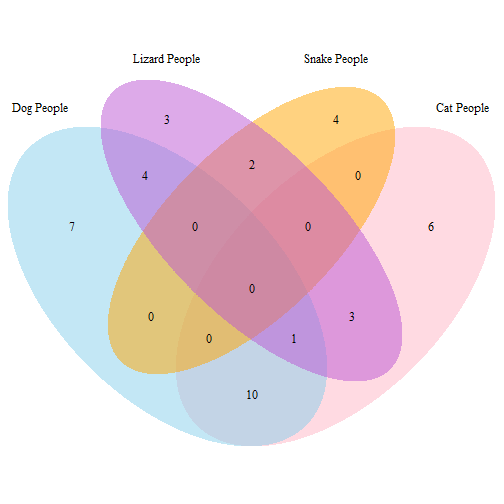``````## (polygon[GRID.polygon.4484], polygon[GRID.polygon.4485], polygon[GRID.polygon.4486], polygon[GRID.polygon.4487], polygon[GRID.polygon.4488], polygon[GRID.polygon.4489], polygon[GRID.polygon.4490], polygon[GRID.polygon.4491], text[GRID.text.4492], text[GRID.text.4493], text[GRID.text.4494], text[GRID.text.4495], text[GRID.text.4496], text[GRID.text.4497], text[GRID.text.4498], text[GRID.text.4499], text[GRID.text.4500], text[GRID.text.4501], text[GRID.text.4502], text[GRID.text.4503], text[GRID.text.4504], text[GRID.text.4505], text[GRID.text.4506], text[GRID.text.4507], text[GRID.text.4508], text[GRID.text.4509], text[GRID.text.4510])
``````

### A different package: venneuler

The venneuler package is a lot cleaner to code, it can take the actual dataset and work out the areas itself. Let's try the 4-set diagram:

``````# install.packages('venneuler')
library(venneuler)

# Using d[-1] to remove 'Participant' column, venneuler doesn't need it.
plot(venneuler(d[-1]))
``````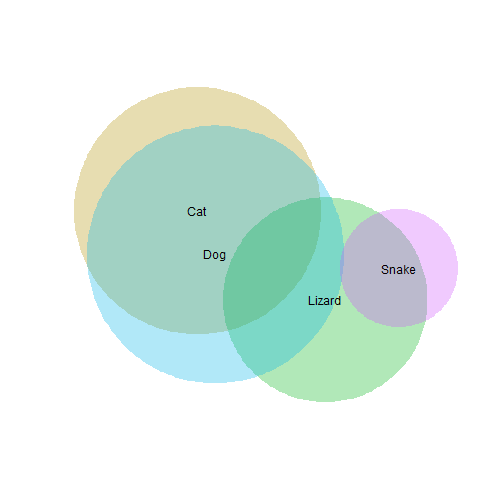That's not right: Snake shouldn't overlap with Dog at all, and Lizard&Cat should overlap more. And there isn't an easy way to get the numbers plotted on there.
To complicate things further, the output changes depending on the format of the input.

``````# Need reshape2 library to reformat the data for venneuler
library(reshape2)

# Melt, so we have a dataframe like 'Participant, Animal, Liked' in long
# form
dSets <- melt(d, id = "Participant")
# Keep Participant and animal ('variable') columns, use 'value' column to
# reduce this to just the set of participants who DO like each animal.
dSets <- (subset(dSets, value == 1))[1:2]

# This is another format that venneuler takes: first column is elements
# (participants) in each set, second column is set names (animals)
plot(venneuler(dSets))
``````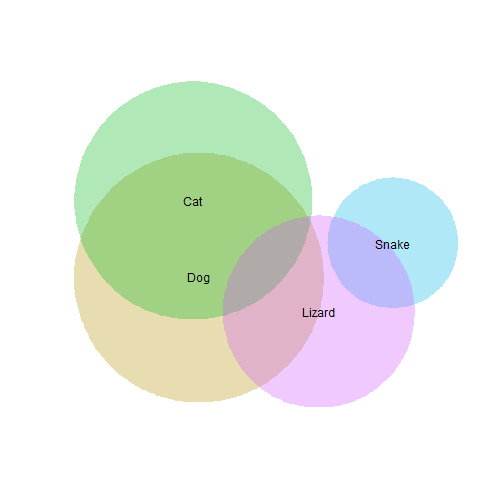And we can even get different results by randomizing the order of the rows in that element-set input.

``````# Randomize dSets by taking a 'sample' of all the row names (gets them in
# random order) and then taking rows from d at those mixed-up row names
dSetsMix <- dSets[sample(rownames(dSets), nrow(dSets)), ]
plot(venneuler(dSetsMix))
``````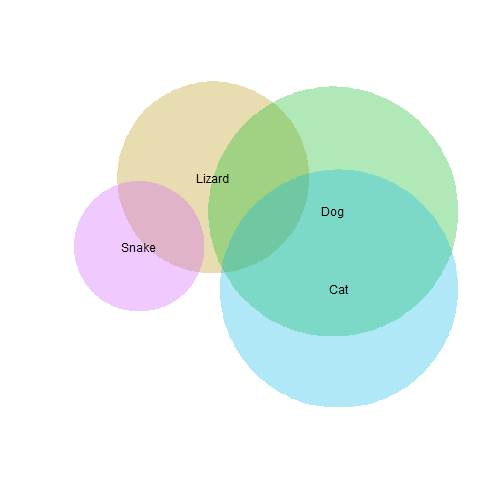``````
# Again
dSetsMix <- dSets[sample(rownames(dSets), nrow(dSets)), ]
plot(venneuler(dSetsMix))
``````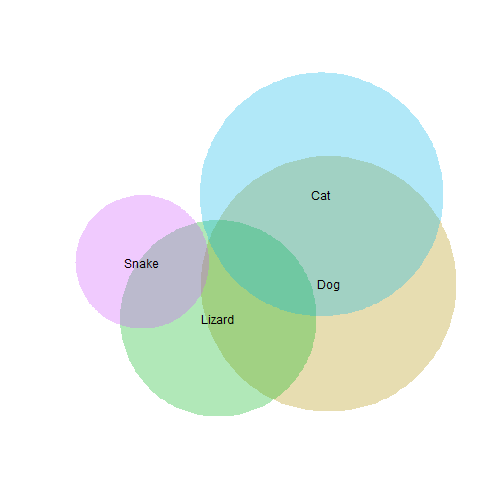``````
# And again
dSetsMix <- dSets[sample(rownames(dSets), nrow(dSets)), ]
plot(venneuler(dSetsMix))
``````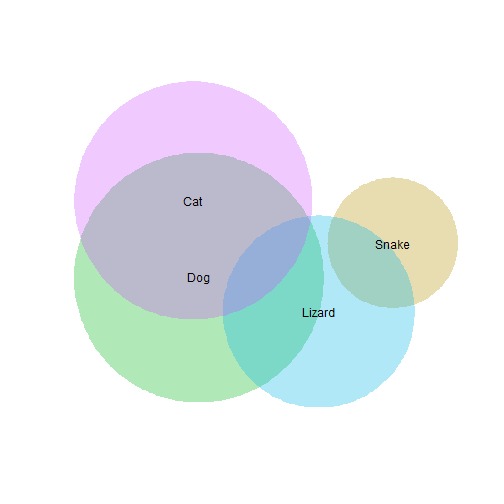The plot gets rearranged a few different ways, and sometimes the snake-dog overlap is fixed, but there's still a problem with the lizard-dog overlap!

And this package doesn't have very solid documentation, so it's difficult to really see what's going on or how we could get it to do what we want.

We tried a few other packages too, and could't find one that would properly reproduce the 4-set diagram that we had drawn by hand.

### A few more examples and links:

Vennerable package is not currently available at CRAN, but still maintained as an R-Forge project. To try installing it, these are the steps that worked for me.

``````install.packages("Vennerable", repos = "http://R-Forge.R-project.org")

# Vennerable needs graph and RBGL, from bioconductor repository
source("http://bioconductor.org/biocLite.R")
biocLite("graph")
biocLite("RBGL")

# Those packages also need reshape and gtools
install.packages("reshape")
install.packages("gtools")
``````

And here is a demo of a Java program that does a pretty good job of fitting diagrams for more than three sets. This is similar to the algorithm used by the venneuler package.